## 8.2.1 Defining a Feedback Plan

Consider a discrete planning problem similar to the ones defined in Formulations 2.1 and 2.3, except that the initial state is not given. Due to this, the cost functional cannot be expressed only as a function of a plan. It is instead defined in terms of the state history and action history. At stage, these are defined as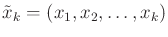(8.1)

and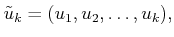(8.2)

respectively. Sometimes, it will be convenient to alternatively refer to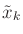as the state trajectory.

The resulting formulation is

Formulation 8..1 (Discrete Optimal Feedback Planning)
1. A finite, nonempty state space.
2. For each state,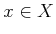, a finite action space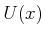.
3. A state transition function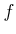that produces a state,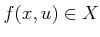, for everyand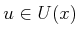. Let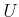denote the union offor all.
4. A set of stages, each denoted by, that begins at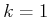and continues indefinitely.
5. A goal set,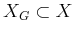.
6. Let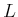denote a stage-additive cost functional,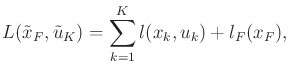(8.3)

in which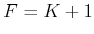.

There is one other difference in comparison to the formulations of Chapter 2. The state space is assumed here to be finite. This facilitates the construction of a feedback plan, but it is not necessary in general.

Consider defining a plan that solves Formulation 8.1. If the initial condition is given, then a sequence of actions could be specified, as in Chapter 2. Without having the initial condition, one possible approach is to determine a sequence of actions for each possible initial state,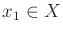. Once the initial state is given, the appropriate action sequence is known. This approach, however, wastes memory. Suppose someis given as the initial state and the first action is applied, leading to the next state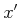. What action should be applied from? The second action in the sequence atcan be used; however, we can also imagine thatis now the initial state and use its first action. This implies that keeping an action sequence for every state is highly redundant. It is sufficient at each state to keep only the first action in the sequence. The application of that action produces the next state, at which the next appropriate action is stored. An execution sequence can be imagined from an initial state as follows. Start at some state, apply the action stored there, arrive at another state, apply its action, arrive at the next state, and so on, until the goal is reached.

It therefore seems appropriate to represent a feedback plan as a function that maps every state to an action. Therefore, a feedback plan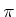is defined as a function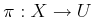. From every state,, the plan indicates which action to apply. If the goal is reached, then the termination action should be applied. This is specified as part of the plan: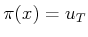, if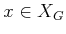. A feedback plan is called a solution to the problem if it causes the goal to be reached from every state that is reachable from the goal.

If an initial state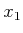and a feedback planare given, then the state and action histories can be determined. This implies that the execution cost, (8.3), also can be determined. It can therefore be alternatively expressed as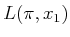, instead of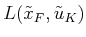. This relies on future states always being predictable. In Chapter 10, it will not be possible to make this direct correspondence due to uncertainties (see Section 10.1.3).

Subsections
Steven M LaValle 2020-08-14